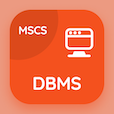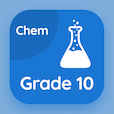Colleges Online Courses

Applied Physics MCQ Questions

Applied Physics MCQs PDF - Chapters

# Applied Physics: Motion and Force Multiple Choice Questions Online p. 9

Learn Applied Physics Motion and Force multiple choice questions and answers, Applied Physics Motion and Force quiz answers PDF to learn Applied Physics worksheets 9 for online courses. Velocity Time Graph MCQs, Applied Physics Motion and Force trivia questions and answers for placement and to prepare for job interview. "Applied Physics Motion and Force MCQ" PDF Book: velocity time graph, uniformly accelerated motion, projectile motion, momentum in physics, physics equations career test for ACT test.

"Area under the velocity-time graph is equal to the" Multiple Choice Questions (MCQ) on applied physics motion and force with choices (v × t), 1/2(v × t), 1/2(v + t), and (v + t) for online bachelor degree programs. Practice velocity time graph quiz questions for jobs' assessment test and online courses for SAT test prep classes.

## MCQs on Applied Physics Motion & Force Quiz

MCQ: Area under the velocity-time graph is equal to the

1/2(V × T)
(V × t)
1/2(V + t)
(V + t)

MCQ: Velocity of the vertically thrown ball with time will be

upwards positive
downwards negative
upwards negative
downwards positive

MCQ: Range of the projectile will be maximum when the angle of the projectile is

30
45
60
90

MCQ: Total momentum of an isolated system

remains constant
decreases
increases
doubled

MCQ: If a body moving with initial, constant velocity of 10 m s-1, accelerates for 2 seconds at a constant rate of 12 m s-2, final velocity of the body will be

30 m s-1
34 m s-1
40 m s-1
45 m s-1